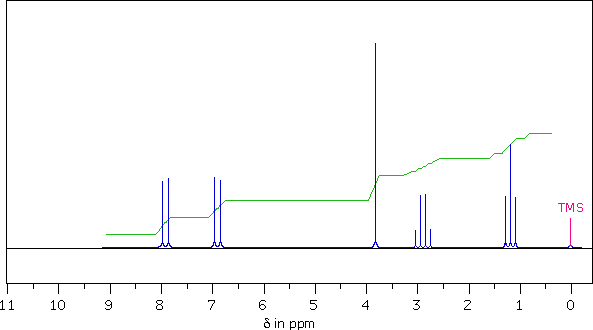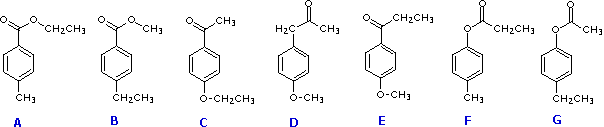加载中…

# 加载中...用户5030268874
• 博客等级：• 博客积分：0
• 博客访问：3,461
• 关注人气：1
• 获赠金笔：0支
• 赠出金笔：0支
• 荣誉徽章：加载中…

## Interpreting a NMR Spectrum(2014-07-06 20:01:17)

### Interpreting a NMR Spectrum

http://orgspectroscopyint.blogspot.in/2014/07/interpreting-nmr-spectrum.htmlInterpreting a NMR Spectrum
The following  1 H nmr spectrum of a C 10 H 12 O 2  Compound Obtained was on a 90 MHz Spectrometer.The 1Hnmr spectrum of the unknown C10H12O2 compound is shown above. Seven structural formulas of some possible candidates for this compound are presented below. After reviewing the chemical shifts, coupling patterns and integration values ​​shown in this spectrum, select the structure most likely to give this spectrum, (A through G)1.   Ignoring the TMS Signal at delta = 0, Discrete groups of Proton How many signals are present in this spectrum?

2.   What is the multiplicity (  s d t q  ) of the Highest field Signal from this Sample?

. 3   .? The Sample has a singlet at delta = 3.8 ppm In Units of Hertz (Hz) How far is this from the TMS Signal Signal . 4   What is Structural Feature Suggested by the singlet at delta = 3.8 ppm? A  CH 3 - C = O      B  -CH 2 -      C  H-O-      D  -O-CH 3 E  C-CH 3 F  H C = C-

5.   From multiplet line separations (Js), which of the Other signals is coupled to the quartet at delta = 2.9 ppm? A  delta = 1.2 ppm      B  delta = 3.8 ppm      C  delta = 6.9 ppm      D  delta = 7.9 ppm

. 6   Using the  Integrator trace  and the Formula of the Sample, assign a Whole Number ratio to the Sample signals as Follows: 7.9 ppm Signal  ;      6.9 ppm Signal  ;      3.8 ppm Signal  ;      2.9 ppm Signal  ;      1.2 ppm SignalStructure Analysis

The Compound's Formula, C 10 H 12 O 2 , has less hydrogen than decane (C 10 H 22 ). The Difference of 10 hydrogens Suggests the Presence of five Double Bonds and / or rings in the structure. Since a benzene Ring has three Double bonds and one ring, and the nmr signals at δ = 7.9 and 6.9 ppm are consistent with aryl hydrogen resonances, we conclude that this compound has a benzene ring.

Integration Indicates the Presence of Two methyl groups, a O-CH 3  at delta = 3.8 ppm, and a C-CH 3  at delta = 1.2 ppm. Course of The Former is a singlet, but the Latter is a triplet (J = 8 Hz) due to Coupling with a-CH 2 -. moiety, which is in Turn Split into a quartet (Signal at delta = 2.9 ppm) From the Chemical shifts, we Conclude there is an ethyl group Bonded to an sp 2  carbon atom hybrid .

If we examine the Structural formulas of the Seven Candidate Compounds, we can Eliminate A, C, F & G Because They do Not have a-O-CH 3  group and Can not account for a singlet at delta = 3.8 ppm. Structure D does Not have an ethyl group, so we are left with B & E as the most likely source of this spectrum.

Not a simple job it is to distinguish B & E by  1 H nmr alone. The Simplicity of the aromatic Proton Region Indicates para-disubstitution of the benzene Ring. If the Two substituents are Similar in nature, the Ring Proton signals Would have Similar Chemical shifts . If the substituents are very different in nature, these signals would also have significantly different chemical shifts. Since the aromatic doublets in this spectrum are separated by 90 Hz (1.0 ppm), we may tentatively select E ​​(oxygen and carbon substituents) over B (two different carbon substituents).

A better means of Distinguishing These Compounds by Infrared Spectroscopy is. Ester carbonyl Stretching Frequencies are usually higher than equivalent KETONE Frequencies by Roughly 25 cm -1 . Also the  13 C nmr carbonyl Signal from Esters (delta =  ca.  170 ppm) is usually Observed at higher field than KETONE carbonyl signals (delta =  ca.  200 ppm).
The spectrum is in fact that of E.1H NMR13 C NMRIR0

• 评论加载中，请稍候...发评论

以上网友发言只代表其个人观点，不代表新浪网的观点或立场。

新浪BLOG意见反馈留言板　电话：4000520066 提示音后按1键（按当地市话标准计费）　欢迎批评指正

新浪公司 版权所有